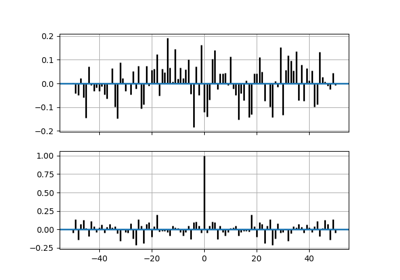# matplotlib.pyplot.xcorr¶

matplotlib.pyplot.xcorr(x, y, normed=True, detrend=<function detrend_none at 0x7f154d2aeca0>, usevlines=True, maxlags=10, *, data=None, **kwargs)[source]

Plot the cross correlation between x and y.

The correlation with lag k is defined as $$\sum_n x[n+k] \cdot y^*[n]$$, where $$y^*$$ is the complex conjugate of $$y$$.

Parameters: xarray-like of length n yarray-like of length n detrendcallable, optional, default: mlab.detrend_nonex and y are detrended by the detrend callable. This must be a function x = detrend(x) accepting and returning an numpy.array. Default is no normalization. normedbool, optional, default: TrueIf True, input vectors are normalised to unit length. usevlinesbool, optional, default: TrueDetermines the plot style. If True, vertical lines are plotted from 0 to the xcorr value using Axes.vlines. Additionally, a horizontal line is plotted at y=0 using Axes.axhline. If False, markers are plotted at the xcorr values using Axes.plot. maxlagsint, optional, default: 10Number of lags to show. If None, will return all 2 * len(x) - 1 lags. lagsarray (length 2*maxlags+1)The lag vector. carray (length 2*maxlags+1)The auto correlation vector. lineArtist added to the axes of the correlation: LineCollection if usevlines is True. Line2D if usevlines is False. bLine2D or NoneHorizontal line at 0 if usevlines is True None usevlines is False. linestyleLine2D property, optionalThe linestyle for plotting the data points. Only used if usevlines is False. markerstr, optional, default: 'o'The marker for plotting the data points. Only used if usevlines is False.

Notes

The cross correlation is performed with numpy.correlate() with mode = "full".

Note

In addition to the above described arguments, this function can take a data keyword argument. If such a data argument is given, the following arguments are replaced by data[<arg>]:

• All arguments with the following names: 'x', 'y'.

Objects passed as data must support item access (data[<arg>]) and membership test (<arg> in data).

## Examples using matplotlib.pyplot.xcorr¶